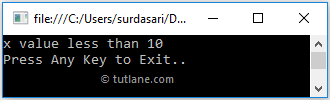# C# If-Else-If Statement with Examples

In c#, if-else-if statement or condition is used to define multiple conditions and execute only one matched condition based on our requirements.

Generally, in c# if statement or if-else statement is useful when we have one condition to validate and execute the required block of statements. If we have multiple conditions to validate and execute only one block of code, then the if-else-if statement is useful in our application.

## Syntax of C# if-else-if Statement

Following is the syntax of defining if-else-if statement in c# programming language.

if (condition_1)
{
// Statements to Execute if condition_1 is True
}
else if (condition_2)
{
// Statements to Execute if condition_2 is True
}
else if (condition_3)
{
// Statements to Execute if condition_3 is True
}
....
....
else{
// Statements to Execute if all conditions are False
}

If you observe the above c# if-else-if statement syntax, we defined multiple conditions to execute the required statements.

Here, the execution of if-else-if statement will start from top to bottom, and as soon as the condition returns true, then the code inside of if or else if block will be executed, and control will come out of the loop.

If none of the conditions return true, then the code inside else block will be executed.

Following is the simple example of using the if-else-if statement in the c# programming language.

int x = 5;
if (x == 10)
{
Console.WriteLine("x value equals to 10");
}
else if (x > 10)
{
Console.WriteLine("x value greater than 10");
}
else
{
Console.WriteLine("x value less than 10");
}

If you observe the above example, the if-else-if statement will start the execution from top to bottom and will check if any condition is matching or not to execute the respective code block. In case if no condition is matching, then the else block will be executed.

## C# If-Else-If Statement Flow Chart Diagram

Following is the flow chart diagram that will represent the process flow of if-else-if statement in the c# programming language.If you observe the above c# if-else-if statement flow chart, if the defined condition is true, then the statements within if condition will be executed; otherwise, it will move to another condition (else-if) to check whether the condition is matching or not. If no conditions are matching, the else block will be executed.

## C# If-Else-If Statement Example

Following is the example of defining the if-else-if statement in c# programming language to execute the block of code or statements based on a Boolean expression.

using System;

namespace Tutlane
{
class Program
{
static void Main(string[] args)
{
int x = 5;
if (x == 10)
{
Console.WriteLine("x value equals to 10");
}
else if (x > 10)
{
Console.WriteLine("x value greater than 10");
}
else
{
Console.WriteLine("x value less than 10");
}
Console.WriteLine("Press Enter Key to Exit..");
}
}
}

If you observe the above code, we defined if-else-if conditions to execute the statements based on defined condition status.

## Output of C# If Else If Statement Example

When we execute the above c# program, we will get the result as shown below.If you observe the above result, all defined conditions are failing due to that it executed an else block of statements and printed the required statement in the console window.

This is how we can use the if-else-if statement in the c# programming language to execute the block of code or statements based on our requirements.